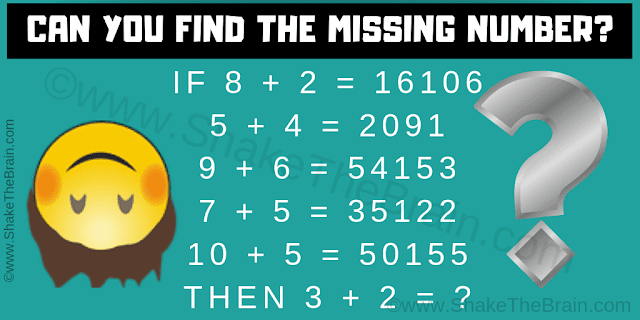This is the high IQ Intelligence Test which will challenge your mind. In this intelligence test, your challenge is to find the hidden logic used in the given logical equations. Study the given logical number equations very carefully to decode the logical reasoning used in these equations and then find the value of the missing number.Can you solve this High IQ Intelligence Test?
The answer to this "High IQ Intelligence Test", can be viewed by clicking on the answer button.

Unknown said...

3+2 would have to equal 566

Rajesh Kumar said...

Unknown said...

3+2 would be 651

Unknown said...

651, do you ever consider puzzles with no answers so people have to comment to find out if they are correct?

Unknown said...

3+2=651 This is because the last digit 1,was obtained by subtracting 2 from 3 ,got the second digit 5 by adding the two numbers which are 3 and 2 and the first digit 6 by multiplying 3 and 2

Unknown said...

It was very easy is solved it the answer is
3*2 = 6
3+2 = 5
3-2. =1
651
I highly doubt this was a high iq question
Are there any harder ones

Unknown said...

651